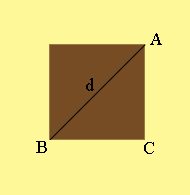SEARCH HOMEMath Central Quandaries & QueriesQuestion from Patricia: I am putting in a new bathroom fan. I am wondering if a new light with a 15 inch diameter will cover the existing square hole which is 12 by 12 inches? If the existing hole is 11 1/2 by 11 1/2 inches? Also, if the 15 inch diameter does not cover the 12 by 12 hole, what size diameter would? Thank you.Hi Patricia,

It is amazing g to me how many times Pythagoras Theorem is what you need to solve everyday problems.I drew a piece of your bathroom wall with the existing hole and labeled three of the vertices. You know that $|BC| = 12$ inches, $|CA| = 12$ inches and I let $|AB| = d$ inches. Pythagoras Theorem says that

$|BC|^2 + |CA|^2 = d^2$

so

$12^2 + 12^2 = d^2$

and thus $d^2 = 288$ or $d = 17.0$ inches and hence your 15 inch diameter cover won't work. If the hole is 11.5 inches by 11.5 inches then I get $d^2 = 2 \times 11.5^2$ or $d = 16.3$ inches so that won't work either.

I hope this helps,
PennyMath Central is supported by the University of Regina and the Imperial Oil Foundation.